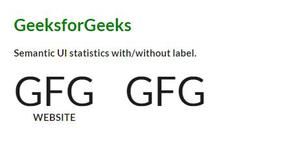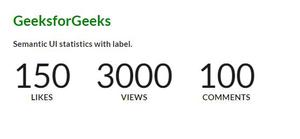Open in App
Not now

# Semantic-UI Statistics Content Label

• Last Updated : 06 Feb, 2022

Semantic UI is a UI framework that offers a bunch of UI tools to style web pages. Representation of content in UI  is made better through this framework. It has a bunch of components for users to design web pages. One of them is “Statistics”. It is used to magnify particular data on a web page. There are different ways of content representation in a statistic. Let us see the label content statistics.

Semantic UI Statistics Label Content contains a label that provides a reference for the value. Suppose the user wants to highlight data on UI he also needs to indicate in some cases, which part of content he is referring to. That’s when label content helps us out. There may be a bunch of data to be used as statistics. To differentiate among them labels can be put on each of them. We will design a UI showing the label content statistics.

Semantic-UI Statistics Content Label Class:

• label: This class is used to provide context for the presented value.

Syntax:

```<div class="ui statistic">
<div class="value">
....
</div>
<div class="label">
....
</div>
</div>```

Below example illustrates the Semantic-UI Statistics Content Label:

Example 1: This example demonstrates the label representation using the label class.

## HTML

 ` ` `<``html``> ` ` `  `<``head``> ` `    ``<``link` `href``= ` `"https://cdnjs.cloudflare.com/ajax/libs/semantic-ui/2.4.1/semantic.min.css"`  `          ``rel``=``"stylesheet"` `/> ` ` ` ` `  `<``body``> ` `    ``<``div` `class``=``"ui container"``> ` `        ``<``h2` `style``=``"color:green"``>GeeksforGeeks ` `        ``<``b``> ` `            ``<``p``>Semantic UI statistics with/without label. ` `        `` ` `        ``<``div` `class``=``"ui statistics"``> ` `            ``<``div` `class``=``"statistic"``> ` `                ``<``div` `class``=``"value"``> ` `                    ``GFG ` `                `` ` `                ``<``div` `class``=``"label"``> ` `                    ``Website ` `                `` ` `            `` ` `            ``<``div` `class``=``"statistic"``> ` `                ``<``div` `class``=``"value"``> ` `                    ``GFG ` `                `` ` `            `` ` `        `` ` `    `` ` ` ` ` `  ``

Output:Semantic-UI Statistics Content Label

Example 2: This example demonstrates the bunch of data each having a label representation using the label class.

## HTML

 ` ` `<``html``> ` ` `  `<``head``> ` `    ``<``link` `href``= ` `"https://cdnjs.cloudflare.com/ajax/libs/semantic-ui/2.4.1/semantic.min.css"`  `          ``rel``=``"stylesheet"` `/> ` ` ` ` `  `<``body``> ` `    ``<``div` `class``=``"ui container"``> ` `        ``<``h2` `style``=``"color:green"``>GeeksforGeeks ` `        ``<``b``> ` `            ``<``p``>Semantic UI statistics with label. ` `        `` ` `        ``<``div` `class``=``"ui statistics"``> ` `            ``<``div` `class``=``"statistic"``> ` `                ``<``div` `class``=``"value"``> ` `                    ``150 ` `                `` ` `                ``<``div` `class``=``"label"``> ` `                    ``Likes ` `                `` ` `            `` ` `            ``<``div` `class``=``"statistic"``> ` `                ``<``div` `class``=``"value"``> ` `                    ``3000 ` `                `` ` `                ``<``div` `class``=``"label"``> ` `                    ``Views ` `                `` ` `            `` ` `            ``<``div` `class``=``"statistic"``> ` `                ``<``div` `class``=``"value"``> ` `                    ``100 ` `                `` ` `                ``<``div` `class``=``"label"``> ` `                    ``Comments ` `                `` ` `            `` ` `        `` ` `    `` ` ` ` ` `  ``

Output:Semantic-UI Statistics Content Label

My Personal Notes arrow_drop_up
Related Articles